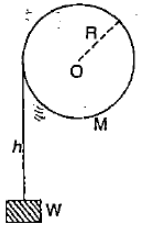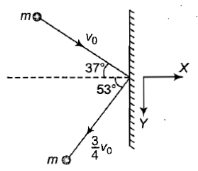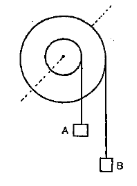In the following figure, a weight W is attached to a string wrapped round a solid cylinder of mass M mounted on a frictionless horizontal axle at O.

If the weight starts from rest and falls a distance h, then its speed at that instant is(1) Proportional to R

(2) Proportional to $\frac{1}{R}$

(3) Proportional to $\frac{1}{{R}^{2}}$

(4) Independent of R

Concept Questions :-

Torque
High Yielding Test Series + Question Bank - NEET 2020

Difficulty Level:

A solid cylinder rolls down an inclined plane that has friction sufficient to prevent sliding. The ratio of rotational energy to total kinetic energy is

1.  $\frac{1}{2}$

2.  $\frac{1}{3}$

3.  $\frac{2}{3}$

4.  $\frac{3}{4}$

Concept Questions :-

Rolling motion
High Yielding Test Series + Question Bank - NEET 2020

Difficulty Level:

A swimmer while jumping into water from a height easily forms a loop in the air, if

1. He pulls his arms and legs in

2. He spreads his arms and legs

3. He keeps himself straight

4. None of the above

Concept Questions :-

Angular momentum
High Yielding Test Series + Question Bank - NEET 2020

Difficulty Level:

Assertion : A ladder is more apt to slip when you are high up on it than when you just begin to climb

Reason : At the high point on a ladder, the torque is large and on climbing up the torque is small

1. If both the assertion and the reason are true and the reason is a correct explanation of the assertion
2. If both the assertion and reason are true but the reason is not a correct explanation of the assertion
3. If the assertion is true but the reason is false
4. If both the assertion and reason are false

Concept Questions :-

Torque
High Yielding Test Series + Question Bank - NEET 2020

Difficulty Level:

An inclined plane makes an angle of $30°$ with the horizontal. A solid sphere rolling down this inclined plane from rest without slipping has a linear acceleration equal to

1.  $\frac{g}{3}$

2.  $\frac{5g}{7}$

3.  $\frac{2g}{3}$

4.  $\frac{5g}{14}$

Concept Questions :-

Rolling motion
High Yielding Test Series + Question Bank - NEET 2020

Difficulty Level:

Which of the following statements is correct?

1.   Kinetic energy and momentum both are conserved in all types of collisions

2.   Total kinetic energy is not conserved but momentum is conserved in inelastic COI-
lisions

3.  Momentum is conserved In elastic collisions but .not in inelastic collisions

4.  Total kinetic energy is conserved in inelastic collisions but momentum is not conserved in elastic collisions

Concept Questions :-

Linear momentum
High Yielding Test Series + Question Bank - NEET 2020

Difficulty Level:

A ball of mass m moving with velocity ${v}_{0}$ collides a wall as shown in the figure. After impact, it rebounds with a velocity $\frac{3}{4}{v}_{0}$. The impulse acting on ball during impact is1.

2.

3.

4.  None of these

Concept Questions :-

Linear momentum
High Yielding Test Series + Question Bank - NEET 2020

Difficulty Level:

The figure shows a small wheel fixed coaxially on a bigger one of double the radius. The system rotates about the common axis. The strings supporting A and B do not slip on the wheels. If x and y be the distances traveled by A and B in the same time interval, then

1.  x-2y

2.  x = y

3.  y=2x

4.   none of theseConcept Questions :-

Rotation motion : Introduction
High Yielding Test Series + Question Bank - NEET 2020

Difficulty Level:

If a gymnast, sitting on a rotating stool, with his arms outstretched, suddenly lowers his hands

1.  the angular velocity increases

2.  his moment of inertia increases

3.  the angular velocity stays constant

4.  the angular momentum increases

Concept Questions :-

Angular momentum
High Yielding Test Series + Question Bank - NEET 2020

Difficulty Level:

Moment of inertia of a uniform circular disc about a diameter is I. Its moment of inertia about an axis perpendicular to its plane and passing through a point on its rim will be

1.  5 I

2.  3 I

3.  6 I

4.  4 I

Concept Questions :-

Moment of inertia